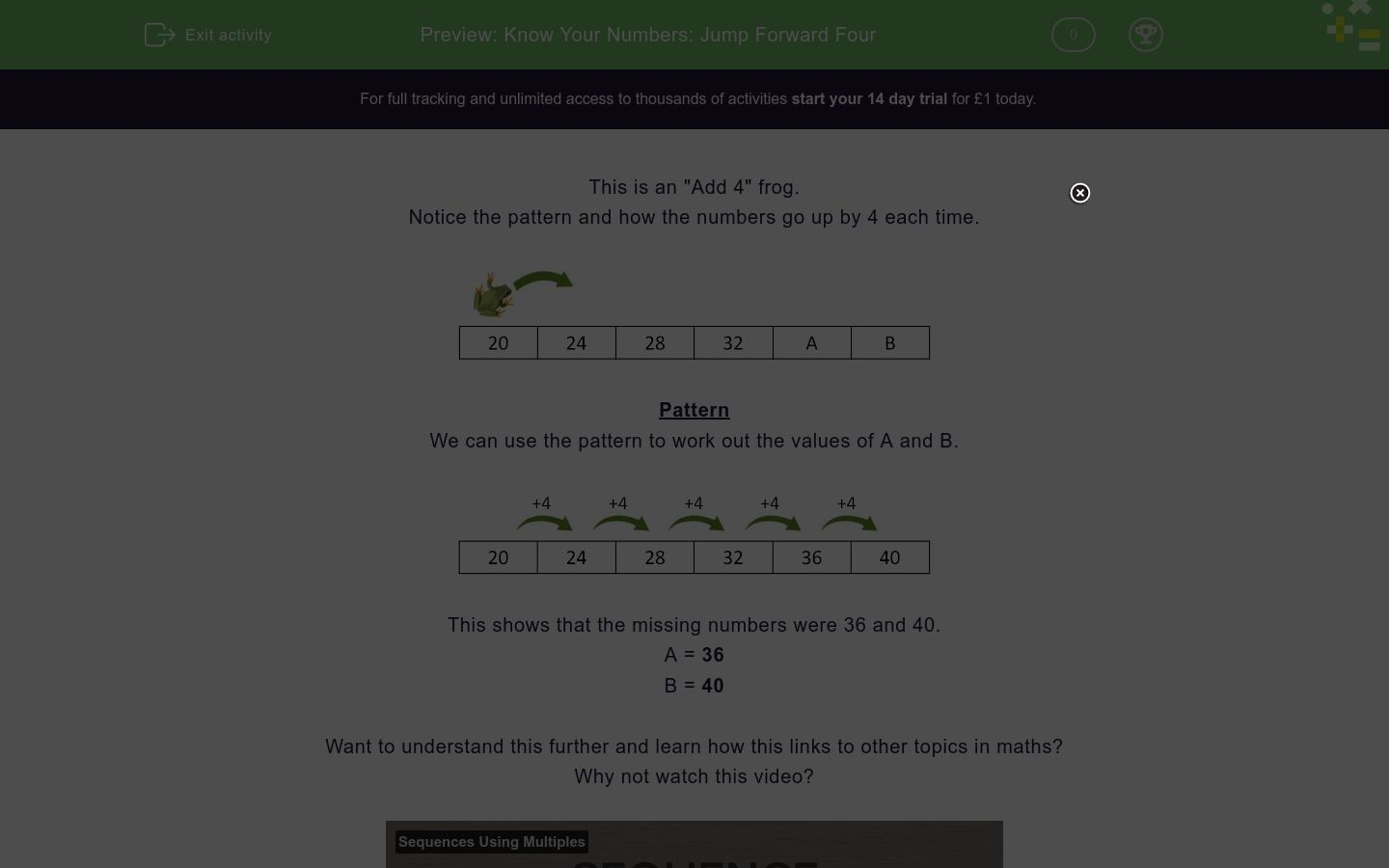# Know Your Numbers: Jump Forward Four

In this worksheet, students find the missing numbers in a sequence that goes up in fours.Key stage:  KS 2

Curriculum topic:   Number: Number and Place Value

Curriculum subtopic:   Count in Multiples (4, 8, 50 and 100)

Difficulty level:### QUESTION 1 of 10

This is an "Add 4" frog.

Notice the pattern and how the numbers go up by 4 each time.Pattern

We can use the pattern to work out the values of A and B.This shows that the missing numbers were 36 and 40.

A = 36

B = 40

Want to understand this further and learn how this links to other topics in maths?
Why not watch this video?

This is a "Take Away 4" frog.

Work out the values of A and B.28 24 20 16 A B
 13 18 12 8 A B

This is an "Add 4" frog.

Work out the values of A and B.A 21 25 29 33 B
 33 24 17 37 A B

This is an "Add 4" frog.

Work out the values of A and B.A 19 23 27 B 35
 31 17 15 32 A B

This is an "Add 4" frog.

Work out the values of A and B.14 18 A 26 B 34
 32 30 22 23 A B

This is an "Add 4" frog.

Work out the values of A and B.10 14 A 22 B 30
 18 26 20 25 A B

This is an "Add 4" frog.

Work out the values of A and B.1 A 9 13 B 21
 5 6 17 19 A B

This is an "Add 4" frog.

Work out the values of A and B.5 9 13 A 21 B
 15 26 17 25 A B

This is an "Add 4" frog.

Work out the values of A and B.4 A 12 16 20 B
 24 6 8 26 A B

This is an "Add 4" frog.

Work out the values of A and B.8 A B 20 24 28
 16 14 12 18 A B

This is an "Add 4" frog.

Work out the values of A and B.A 12 16 20 24 B
 6 8 26 28 A B
• Question 1

This is a "Take Away 4" frog.

Work out the values of A and B.28 24 20 16 A B
 13 18 12 8 A B
EDDIE SAYS
20... 16... 12... 8
• Question 2

This is an "Add 4" frog.

Work out the values of A and B.A 21 25 29 33 B
 33 24 17 37 A B
EDDIE SAYS
17... 21... 25... 29... 33... 37
• Question 3

This is an "Add 4" frog.

Work out the values of A and B.A 19 23 27 B 35
 31 17 15 32 A B
• Question 4

This is an "Add 4" frog.

Work out the values of A and B.14 18 A 26 B 34
 32 30 22 23 A B
EDDIE SAYS
14... 18... 22... 26... 30
• Question 5

This is an "Add 4" frog.

Work out the values of A and B.10 14 A 22 B 30
 18 26 20 25 A B
EDDIE SAYS
10... 14... 18... 22... 26
• Question 6

This is an "Add 4" frog.

Work out the values of A and B.1 A 9 13 B 21
 5 6 17 19 A B
EDDIE SAYS
1... 5... 9... 13... 17
• Question 7

This is an "Add 4" frog.

Work out the values of A and B.5 9 13 A 21 B
 15 26 17 25 A B
EDDIE SAYS
13... 17... 21... 25
• Question 8

This is an "Add 4" frog.

Work out the values of A and B.4 A 12 16 20 B
 24 6 8 26 A B
EDDIE SAYS
4... 8... 12... 16... 20... 24
• Question 9

This is an "Add 4" frog.

Work out the values of A and B.8 A B 20 24 28
 16 14 12 18 A B
EDDIE SAYS
8... 12... 16... 18
• Question 10

This is an "Add 4" frog.

Work out the values of A and B.A 12 16 20 24 B
 6 8 26 28 A B
EDDIE SAYS
8... 12... 16... 20... 24... 28
---- OR ----

Sign up for a £1 trial so you can track and measure your child's progress on this activity.

### What is EdPlace?

We're your National Curriculum aligned online education content provider helping each child succeed in English, maths and science from year 1 to GCSE. With an EdPlace account you’ll be able to track and measure progress, helping each child achieve their best. We build confidence and attainment by personalising each child’s learning at a level that suits them.

Get started